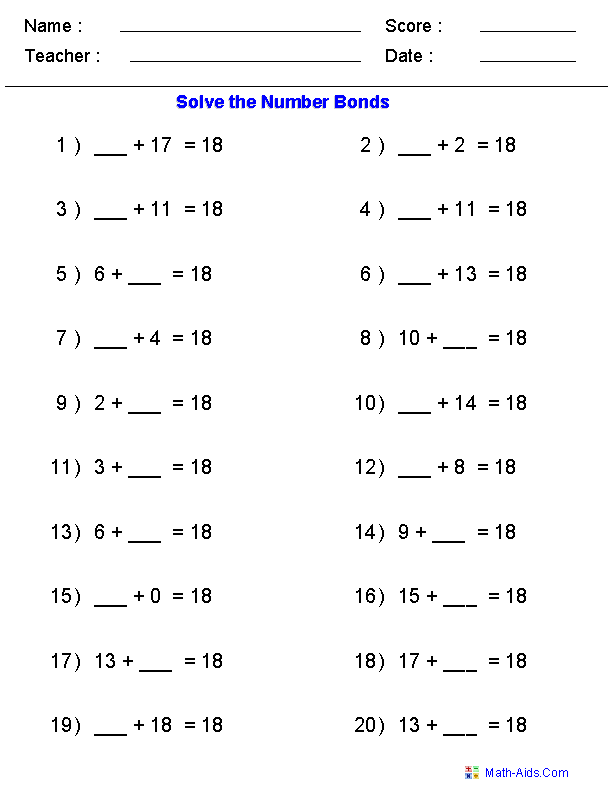#Number Bonds Worksheets

## Printable Number Bonds Worksheets

These Number Bonds Worksheets are great for testing children in their ability to solve number bonds problems for a given sum. A number bond is a mental picture of the relationship between a number and the parts that combine to make it. The concept of number bonds is very basic, an important foundation for understanding how numbers work. A whole thing is made up of parts. If you know the parts, you can put them together (add) to find the whole. If you know the whole and one of the parts, you take away the part you know (subtract) to find the other part.

Number bonds let children see the inverse relationship between addition and subtraction. Subtraction is not a totally different thing from addition; they are mirror images. To subtract means to figure out how much more you would have to add to get the whole thing.

These Number Bonds Worksheets are a great resource for children in Kindergarten, 1st Grade, and 2nd Grade.

Click here for a Detailed Description of all the Number Bonds Worksheets.

## Quick Link for All Number Bonds Worksheets

Click the image to be taken to that Number Bonds Worksheet.

#### Number Bonds WorksheetsHorizontal Format#### Number Bonds WorksheetsTree FormatRecommended Videos

## Detailed Description for All Number Bonds Worksheets

Number Bonds Worksheets - Horizontal Format
These Number Bonds Worksheets are great for testing children in their ability to solve number bonds problems for a given sum. Number Bonds are missing number addition problems that all have the same sum. You may select from 5 to 99 for the sum to be used in the problems. These Number Bonds Worksheets allow you to select the number of problems page and are in a horizontal format.

Number Bonds Worksheets - Tree Format
These Number Bonds Worksheets are great for testing children in their ability to solve number bonds problems for a given sum. Number Bonds are missing number addition problems that all have the same sum. You may select from 5 to 99 for the sum to be used in the problems. These Number Bonds Worksheets will produce 12 problems per page in a tree format.